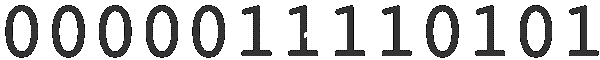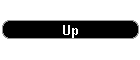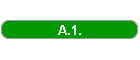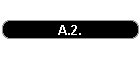Alternate method to fit some experimental z=f(x,y) data, with unknown analytical comportement, by an empirical function (a surface). What is (I suppose) new, the empirical function, which one is a superposition of some functions, non continuos in derivatives, not easy to calculate by hand, but easy for a computer. The component functions are centered in nodes on xy-surface, actually 3*3=9. The small, old program [plane_1.ZIP] and [plane_2.ZIP], containing a lot of bugs, can be used to test the method. Extract both in the same directory and install.Starting the program we see two small form windows. In the window  "MainR1", we can choose between two ways of introducing the input data. Pressing the "DateKEYB" button, it appears a spreadsheet where we can insert a set of data. The spreesheet input is a number of rows of XM, YM, ZM triplets, the experimental data. Selecting one by one the cells of a row, by a click, introducing the corresponding values and validating the row by an ENTER, we can fill this input. Actually they are necessary at least of NM = 9 input points. The program automatically updates the NM and the XMIN, XMAX, YMIN, YMAX, ZMIN, ZMAX (the minimum minimorum and the maximum maximorum of input x, y, z) values, necessary later. From this point the procedure is the same as for the second way. The second way begins with a click on the dropdown combo, which one presents a lot of files with different input and output data. Corresponding, they are two kind of files in the dropdown combo. The ???????i.dat and the ???????c.dat files. The "i" files contains just the different input data, the "c" files the input and the calculated values (the precision indices of the fit, the parameters of the empirical functions...). From the dropdown combo we can choose a file with some test data and with a click on DateDISK start the procedure. Again appears the spreadsheet, now with the data from the selected data file..     The format of the input file is, line by line:     A line with     XMIN, XMAX, NX, NVX    #####.##, #####.##, ##, ##     where NX-1 is the number of subintervals on the x axis     and NXV the "node-sections" on the x axis (actually don't work)     similar a line for the y values:     YMIN, YMAX, NY, NVY    #####.##, #####.##, ##, ##     The NX*NY points divides in (NX-1)*(NY-1) rectangles the xy-plane.     The next line contains the number of input, "experimental", values     NM     ####     followed by the triplets, NM times*3 lines     XM     #####.##        YM     #####.##     ZM     #####.## A click on the "Retea" button, followed by a click on the "Mcmmp" button, some patience, and we can see the results, mainly in the second forms window, "Log 2". In this window appears the precision indices:     OMEGA=[sum of (ZM-ZC)**2 over NM] / ( NM- 9 )     where ZC are by the empirical function calculated values     with the following remark:     IF (NM-9)=0 THEN                           OMEGA=999                           PZO=999     ELSE                           PZO= 0.6745*SQRT( OMEGA) The chi-square value is calculated as:     HI=sum of (ZM-ZC)**2 / ZC over NM Remark: - we can edit the input data of any "i" file - we can use the "o" files as input files At the other hand, we can use the spreadsheet window to calculate some particular values, introducing X, Y and then clicking in Z for the calculated value. If we want, we can see the results graphically, clicking on the "GraficR" button from the spreadsheets window. The red bars are the z-distances of the input values up from the calculated surface values, the blue are the same for that input values "under" the surface. The black lines sketchs the empirical surface. The buttons "JOS", "STANDARD", "SUS" makes visible/invisible the red, black and blue points. For better visibility the figure can be rotated by left or right clicks on the buttons "RotireY" and "RotireZ", around the fictiv y and z axis. Attention! On the first click on the "Grafic" window reappears the "MainR" window where we can save the calculated data. With a new click in the graphics window we can continue the inspection. After saving the output data, we can start again with new data in the "MainR" window. Attention! Please don't close the "old" spreadsheet (Tabela x) and graphics (Grafic xxx) windows, here is a bug. In that time, in a schizofrenic tentative, I have tried to develop my "big" method" and to learn the CA Realizer 2, simultaneously. To do (as I have learned from the Linux fans): - to compare the method with the similars, concerning the speed, the efficiency (precision/number of parameters) - to generalize for different divisions in rectangles and, why not, triangles, or other plane figures - to generalize for other dimensions - to introduce the eventually knowed restrictions, slops etc. - to replace my simplest least-square fit with other, sophisticated one, maximum likelyhood methods. Enjoy and don't blame me to much for the bugs !